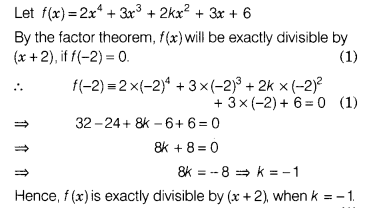# What value of k,the polynomial (2x4 + 3x3 + 2kx2 + 3x + 6)is exactly divisible by (x + 2)?

For what value of k, the polynomial (2x4 + 3x3 + 2kx2 + 3x + 6) is exactly divisible by (x + 2)?